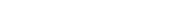# 详解python的二元算术运算

【摘要】家对我解读属性访问的博客文章反应热烈，这启发了我再写一篇关于 python 有多少语法实际上只是语法糖的文章。在本文中，我想谈谈二元算术运算。<pre> `>>> def sub(): a - b... >>> import dis>>> dis.dis(sub)  1           0 LOAD_GLOBAL              0 (a)              2 LOAD_GLOBAL              1 (b)              4 BINARY_SUBTRACT              6 POP_TOP              8 LOAD_CONST               0 (None)             10 RETURN_VALUE`

````case TARGET(BINARY_SUBTRACT): {    PyObject *right = POP();    PyObject *left = TOP();    PyObject *diff = PyNumber_Subtract(left, right);    Py_DECREF(right);    Py_DECREF(left);    SET_TOP(diff);    if (diff == NULL)    goto error;    DISPATCH();}`
```

1、__sub__()方法

````# 通过调用__sub__()实现减法 def sub(lhs: Any, rhs: Any, /) -> Any:    """Implement the binary operation `a - b`."""    lhs_type = type(lhs)    try:        subtract = _mro_getattr(lhs_type, "__sub__")    except AttributeError:        msg = f"unsupported operand type(s) for -: {lhs_type!r} and {type(rhs)!r}"        raise TypeError(msg)    else:        return subtract(lhs, rhs)`
```

2、让右侧使用__rsub__()

3、不关心类型

````# 减法的实现，其中表达式的左侧和右侧均可参与运算_MISSING = object()def sub(lhs: Any, rhs: Any, /) -> Any:        # lhs.__sub__        lhs_type = type(lhs)        try:            lhs_method = debuiltins._mro_getattr(lhs_type, "__sub__")        except AttributeError:            lhs_method = _MISSING        # lhs.__rsub__ (for knowing if rhs.__rub__ should be called first)        try:            lhs_rmethod = debuiltins._mro_getattr(lhs_type, "__rsub__")        except AttributeError:            lhs_rmethod = _MISSING        # rhs.__rsub__        rhs_type = type(rhs)        try:            rhs_method = debuiltins._mro_getattr(rhs_type, "__rsub__")        except AttributeError:            rhs_method = _MISSING        call_lhs = lhs, lhs_method, rhs        call_rhs = rhs, rhs_method, lhs        if lhs_type is not rhs_type:            calls = call_lhs, call_rhs        else:            calls = (call_lhs,)        for first_obj, meth, second_obj in calls:            if meth is _MISSING:                continue            value = meth(first_obj, second_obj)            if value is not NotImplemented:                return value        else:            raise TypeError(                f"unsupported operand type(s) for -: {lhs_type!r} and {rhs_type!r}"            )`
```

4、子类优先于父类

````# Python中减法的完整实现_MISSING = object()def sub(lhs: Any, rhs: Any, /) -> Any:        # lhs.__sub__        lhs_type = type(lhs)        try:            lhs_method = debuiltins._mro_getattr(lhs_type, "__sub__")        except AttributeError:            lhs_method = _MISSING        # lhs.__rsub__ (for knowing if rhs.__rub__ should be called first)        try:            lhs_rmethod = debuiltins._mro_getattr(lhs_type, "__rsub__")        except AttributeError:            lhs_rmethod = _MISSING        # rhs.__rsub__        rhs_type = type(rhs)        try:            rhs_method = debuiltins._mro_getattr(rhs_type, "__rsub__")        except AttributeError:            rhs_method = _MISSING        call_lhs = lhs, lhs_method, rhs        call_rhs = rhs, rhs_method, lhs        if (            rhs_type is not _MISSING  # Do we care?            and rhs_type is not lhs_type  # Could RHS be a subclass?            and issubclass(rhs_type, lhs_type)  # RHS is a subclass!            and lhs_rmethod is not rhs_method  # Is __r*__ actually different?        ):            calls = call_rhs, call_lhs        elif lhs_type is not rhs_type:            calls = call_lhs, call_rhs        else:            calls = (call_lhs,)        for first_obj, meth, second_obj in calls:            if meth is _MISSING:                continue            value = meth(first_obj, second_obj)            if value is not NotImplemented:                return value        else:            raise TypeError(                f"unsupported operand type(s) for -: {lhs_type!r} and {rhs_type!r}"            )`
```

````# 一个创建闭包的函数，实现了二元运算的逻辑_MISSING = object()def _create_binary_op(name: str, operator: str) -> Any:    """Create a binary operation function.    The `name` parameter specifies the name of the special method used for the    binary operation (e.g. `sub` for `__sub__`). The `operator` name is the    token representing the binary operation (e.g. `-` for subtraction).    """    lhs_method_name = f"__{name}__"    def binary_op(lhs: Any, rhs: Any, /) -> Any:        """A closure implementing a binary operation in Python."""        rhs_method_name = f"__r{name}__"        # lhs.__*__        lhs_type = type(lhs)        try:            lhs_method = debuiltins._mro_getattr(lhs_type, lhs_method_name)        except AttributeError:            lhs_method = _MISSING        # lhs.__r*__ (for knowing if rhs.__r*__ should be called first)        try:            lhs_rmethod = debuiltins._mro_getattr(lhs_type, rhs_method_name)        except AttributeError:            lhs_rmethod = _MISSING        # rhs.__r*__        rhs_type = type(rhs)        try:            rhs_method = debuiltins._mro_getattr(rhs_type, rhs_method_name)        except AttributeError:            rhs_method = _MISSING        call_lhs = lhs, lhs_method, rhs        call_rhs = rhs, rhs_method, lhs        if (            rhs_type is not _MISSING  # Do we care?            and rhs_type is not lhs_type  # Could RHS be a subclass?            and issubclass(rhs_type, lhs_type)  # RHS is a subclass!            and lhs_rmethod is not rhs_method  # Is __r*__ actually different?        ):            calls = call_rhs, call_lhs        elif lhs_type is not rhs_type:            calls = call_lhs, call_rhs        else:            calls = (call_lhs,)        for first_obj, meth, second_obj in calls:            if meth is _MISSING:                continue            value = meth(first_obj, second_obj)            if value is not NotImplemented:                return value        else:            exc = TypeError(                f"unsupported operand type(s) for {operator}: {lhs_type!r} and {rhs_type!r}"            )            exc._binary_op = operator            raise exc`
```

2020-08-19：修复了当__rsub__() 比 __sub__() 先调用时的规则。 2020-08-22：修复了当类型相同时不调用__rsub__ 的问题；还精简了过渡代码，仅保留开头和结尾代码，这让我轻松些。 2020-08-23：在多数示例中添加了内容。

##### 就业培训申请领取|

# 绑定手机号Python编程各地入口iPhone版